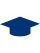polito.it## Time-periodic sensitivity analysis methods for chaotic systemsAndrea Bongiovanni

Time-periodic sensitivity analysis methods for chaotic systems.

Rel. Gaetano Iuso. Politecnico di Torino, Corso di laurea magistrale in Ingegneria Aerospaziale, 2018PDF (Tesi_di_laurea) - Tesi Restricted to: Repository staff only until 5 December 2021 (embargo date). Licenza: Creative Commons Attribution Non-commercial No Derivatives. Download (6MB)
Abstract: A new approach for sensitivity analysis of chaotic dynamical systems is presented. Classical sensitivity analysis methods, used to understand how design and control variables of a given system affect an output of interest, bring to a cumulative error growth if they are applied to a chaotic system with trajectories which are long relative to the predictability time scale of the problem. This occurs because a finite amplitude perturbation in the initial condition leads the finite-time statistical quantities of the observable of interest to diverge exponentially from the reference trajectory. The purpose of this project is to validate a new sensitivity analysis algorithm to obtain a solution that remains bounded over the time interval considered. The method presented here is termed Periodic Shadowing (PS) and solves the linearised sensitivity equations by coupling it to periodic boundary condition in time and allows to compute the gradient of long time statistical quantities. After explaining how this mathematical formulation has been reached, the method will be demonstrated on two low-dimensional chaotic systems of increasing complexity arising from natural convection problems in bounded fluid cells heated from below: the Lorenz system and a model arising from the three-dimensional cells in dissipative Rayleigh–Bénard convection. In particular, we evaluate the sensitivity of the statistical quantities of an observable related to the heat transfer with respect to a physical parameter proportional to the temperature difference between the plates. We focus the attention on the probability distribution of the solution and we analyse how the norm of the solution behaves considering longer time span. We evaluate also the finite-difference approximations of the gradient which show that the Periodic Shadowing method introduces a small bias error. The conjecture about this bias error supports that it could be negligible if higher-dimensional chaotic systems are analysed. The effects of the number of degrees of freedom on this bias is an interesting starting point for future works. Gaetano Iuso 2018/19 Electronic 109 Corso di laurea magistrale in Ingegneria Aerospaziale New organization > Master science > LM-20 - AEROSPATIAL AND ASTRONAUTIC ENGINEERING University of Southampton (REGNO UNITO) UNSPECIFIED http://webthesis.biblio.polito.it/id/eprint/9188Modify record (reserved for operators)